Mohamed Bakrey Mahmoud — Updated On June 23rd, 2022

This article was published as a part of the Data Science Blogathon.

## Introduction on Explainable AI

I love artificial intelligence and I like to delve into it a lot in all or all aspects, and I do the follow-up every day to see what is new in this field. I made the latest update to me. I missed this title and this technology that is before you today, which is one of the newest and rarest technologies that have been worked on recently. In artificial intelligence, at the end of this era, I have been working on the development of this new technology, which is called interpretable artificial intelligence, which works to clarify a new concept, which is how to communicate information better and well to the ordinary person, meaning that it works to make the results more flexible than before. As it works on drawing new, easy-to-understand, and easy-to-flex plans to make the average person work to understand these results in a large and accurate way.

## What is Explainable AI?

Explanatory Artificial Intelligence (XAI) has been created as it is programmed to describe its purpose in explanation and give its accuracy, rationale, and decision-making process in a way that can be understood by the average human. XAI is often discussed in relation to deep learning and plays an important role in the FAT ML model where fairness, accountability, and transparency are in machine learning. And XAI provides a lot of information about how the AI program makes a certain decision about something, as it is taken and followed ways to detect it, namely: Strengths and weaknesses of the program used.

Establish those specific criteria that the program uses to arrive at a specific and accurate decision.
Why does the program make a particular decision rather than those alternatives it depends on?
Establish what is called the appropriate level of confidence for various types of decisions.
What are the types of errors that the program has to clarify and display?

## Understand in Depth About Explainable AI

First of all, we must understand what XAI is and why this technology is needed. Hence, AI algorithms often act as “black boxes” that come in and provide the output anyway to understand their inner workings. Where the goal of Xai is to make the rationale behind producing an algorithm that is understandable to the ordinary person who is not familiar with the subject, making him fully aware of that subject. Hence we can assume that, for example, many AI algorithms are used deep learning in this matter, where the algorithms learn to identify patterns based on the data bloating and the data with large training data. Whereas, deep learning is a neural network approach that simulates the way the brain of normal human beings operates like ours. As with human thought processes, it can be difficult or impossible to determine the extent to which a deep learning algorithm has reached a prediction or decision.

Here, decisions about employment and financial services issues such as credit scores and loan approvals are important and worth explaining. However, no one is likely to be physically harmed (at least immediately) if one of those algorithms gives poor results. But there are many examples where the dire consequences are much more than that.
Hence, deep learning algorithms are increasingly important in health care application use cases such as cancer screening, where it is important for clinicians to understand the basis for algorithm diagnosis. A false negative can mean that the patient is not receiving life-saving treatment. A false positive, on the other hand, may result in a patient receiving expensive treatment when it is most needed and necessary. The level of explanation is essential for radiologists and oncologists seeking to take full advantage of the growing benefits of AI.

## How Does Explainable AI Work?

First, we define and understand what interpretable AI is. Here we will explain how these principles help determine the expected output from XAI, but they do not provide any guidance on how to reach this desired outcome. It may be easy and well to divide XAI into three categories: Where these questions are asked to clarify more and more, and the questions range as follows:

Interpretable data: What data was entered in training the model? Why was this date chosen? How was fairness assessed? Has any effort been made to remove bias?
Interpretable predictions: What features of the model are activated or used to reach certain outputs?
Interpretable algorithms: What individual layers does the model consist of, and how do they lead to the output or prediction?
Looking at the neural network, in particular, interpretable data is the only category that is easy to achieve—at least in principle. Most researchers, or in other words, research leaders put the most emphasis on achieving interpretable predictions and algorithms.
There are two current ways of interpretation:
Proxy modeling: A different type is used where the models are clarified and simplified than using methods such as a decision tree to approximate the actual model. Since it is an approximation, it may differ from the actual model results.
Interpretation design: The forms are designed to be easy to explain. This approach risks reducing the predictive power or overall accuracy of the model.

## What are the Different Types of XAI?

We can work on the classification of XAI under two types:
These models are simple and fast to implement. Algorithms consist of simple computations that ordinary humans can do themselves. Thus, these models explain, and humans can easily understand how these models arrive at a particular decision.
Interpretable AI provides a direct understanding of how AI calculates the required output. There are many rare models that we will mention, which are types of transparent AI. It is important for building trust between people and algorithms because it helps the user to understand how AI works. Interactive AI allows users to interact with AI to work together.
Explainable AI: This type of artificial intelligence is explainable, as it is one of the most important types of XAI because it explains how artificial intelligence is made, how it works, and how to explain those parts that these models work on.
Transparent AI: This type of transparent artificial intelligence is necessary to build trust between people and the algorithms that work to help people work and get many of the tasks and solutions required.
Interactive artificial intelligence: Interactive AI is a type of XAI that allows users to interact with the device in use.

## What are the Features of the Xai Interface?

Features of this interface include here: XAI interfaces depict the output of different data points to explain the relationships between specific features and model predictions. Where users can observe the x and y values of different data points and understand their effect on the absolute error received from the color code to understand it. It makes models easier and clearer in the ideas they present to normal people so that they can understand exactly how to interact with that feature that works in this figure. XAI interfaces visualize the output of different data points to explain the relationships between specific features and model predictions.

## How does XAI Serve AI?

When we saw that artificial intelligence is more widely used in our daily lives, from here we went to an important point, which is the ethics of artificial intelligence. However, the increasing complexity of advanced AI models and the lack of easiness raise doubts about these models. Without understanding them, humans cannot decide whether these AI models are socially useful, trustworthy, safe, and fair. Thus, AI models need to follow specific ethical guidelines. Gartner combines the ethics of artificial intelligence into five main components:

1. Clarity and transparency
2. Human-centered and socially beneficial
3. Exhibition.
4. Safe and secure.
5. Responsible.
One of Xai’s primary goals is to help AI models serve these five components. Where humans need a deep understanding of AI models to understand whether they follow these components or not. Humans cannot trust an AI model that does not know how that model works. By understanding how these models work, humans can decide whether AI models follow all five of these characteristics.

## What are Explainable AI Advantages?

Here its advantages will be mentioned as Xai aims to explain how to come to specific decisions or recommendations. From there, he explains and helps humans understand why AI behaves in certain ways and builds trust between human models and AI. The important advantages of Xai accumulate in:
• It improves explanation and transparency: Companies can understand organizational models, better understand developments, and see why they behave in certain ways under certain conditions. Even if it’s a black model, humans can use an interface of interpretation to understand how these AI models achieve certain conclusions.
• Faster adoption: As companies can better understand AI models, they can be trusted with more important decisions
• Debugging optimization: When the system is running unexpectedly, Xai can be used to identify the problem and help developers debug the problem.
• Enable audit for regulatory requirements
There are many benefits that include this technology as there are significant commercial benefits for building interpretability in artificial intelligence systems. In addition to helping address stresses like regulation and many other things, and adopting good practices around accountability and ethics, there are great benefits to be gained from being ahead and investing in interpretability in our day.

## Implementation

Import library:

Python Code:

``
`Information about data:`
`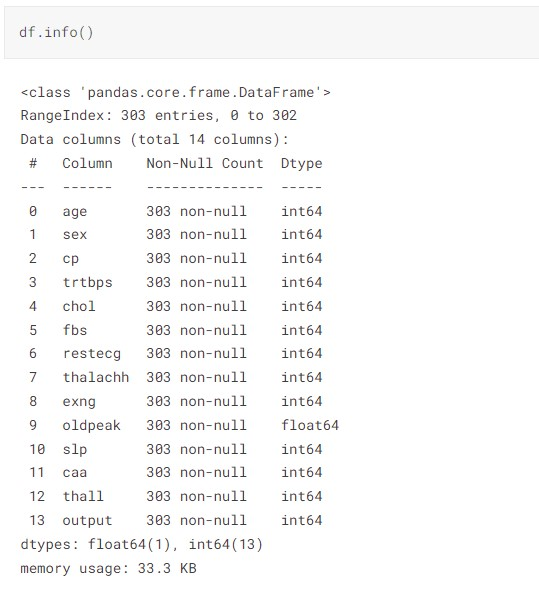`
`df.describe().style.background_gradient(cmap = 'copper')`
`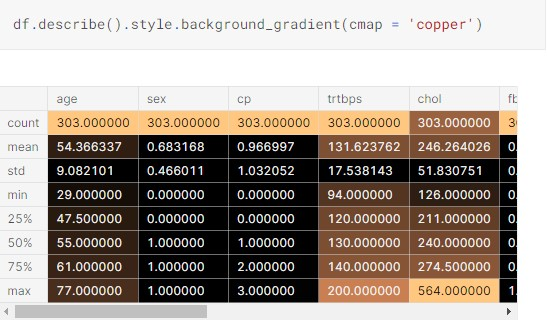`
`df.isna().count()`
`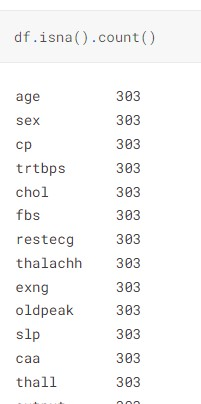`
```fig = ff.create_distplot([df.age],['age'],bin_size=5)
iplot(fig, filename='Basic Distplot')

#Get also the QQ-plot
fig = plt.figure()
res = stats.probplot(df['age'], plot=plt)
plt.show()```
`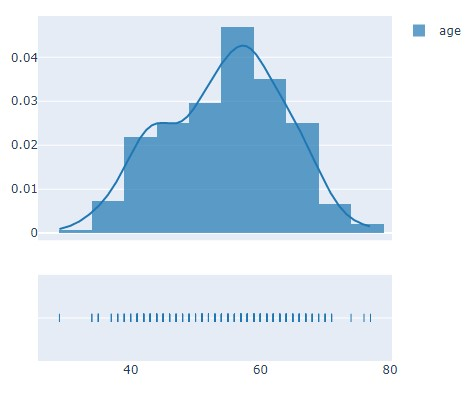`
`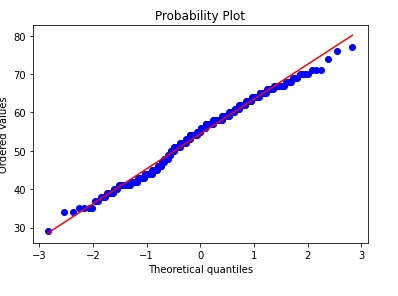`
```print('Heatmap')
plt.figure(figsize=(15,10))
sns.heatmap(df.corr(),annot=True,cmap='coolwarm')```
`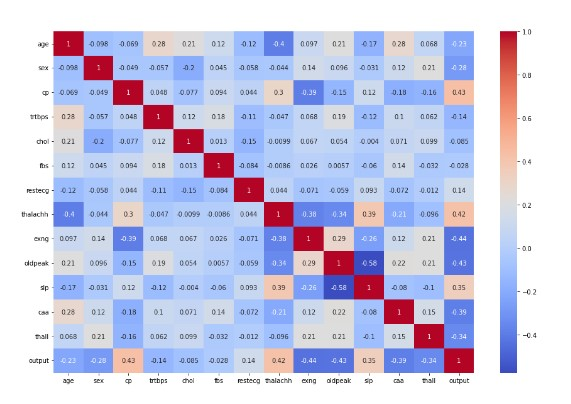`
`Using XAI:`
```!pip install xai
!pip install xai_data
import sys, os
import pandas as pd
import numpy as np
from collections import defaultdict
import matplotlib.pyplot as plt
from sklearn.preprocessing import LabelEncoder, StandardScaler
from sklearn.pipeline import make_pipeline

# Use below for charts in dark jupyter theme

THEME_DARK = False

if THEME_DARK:
# This is used if Jupyter Theme dark is enabled.
# The theme chosen can be activated with jupyter theme as follows:
# >>> jt -t oceans16 -T -nfs 115 -cellw 98% -N  -kl -ofs 11 -altmd
font_size = '20.0'
dark_theme_config = {
"ytick.color" : "w",
"xtick.color" : "w",
"text.color": "white",
'font.size': font_size,
'axes.titlesize': font_size,
'axes.labelsize': font_size,
'xtick.labelsize': font_size,
'ytick.labelsize': font_size,
'legend.fontsize': font_size,
'figure.titlesize': font_size,
'figure.figsize': [20, 7],
'figure.facecolor': "#384151",
'legend.facecolor': "#384151",
"axes.labelcolor" : "w",
"axes.edgecolor" : "w"
}
plt.rcParams.update(dark_theme_config)

sys.path.append("..")

import xai
import xai.data```
`df_groups = xai.imbalance_plot(df, 'age', categorical_cols=categorical_cols)`
`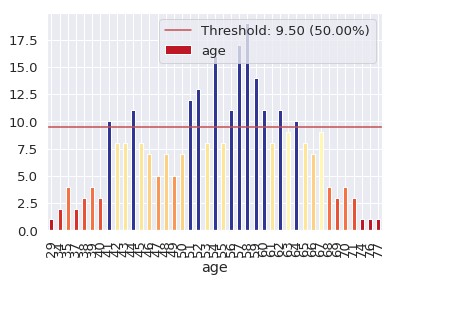`
```proc_df = xai.normalize_numeric(bal_df)
proc_df = xai.convert_categories(proc_df)
x = df.drop("output", axis=1)
y = df["output"]```
```x_train, y_train, x_test, y_test, train_idx, test_idx =
xai.balanced_train_test_split(
x, y, "age",
min_per_group=1,
max_per_group=1,
categorical_cols=categorical_cols)```
```import sklearn
from sklearn.metrics import classification_report, mean_squared_error, roc_curve, auc

from keras.layers import Input, Dense, Flatten,
Concatenate, concatenate, Dropout, Lambda
from keras.models import Model, Sequential
from keras.layers.embeddings import Embedding

def build_model(X):
input_els = []
encoded_els = []
dtypes = list(zip(X.dtypes.index, map(str, X.dtypes)))
for k,dtype in dtypes:
input_els.append(Input(shape=(1,)))
if dtype == "int8":
e = Flatten()(Embedding(X[k].max()+1, 1)(input_els[-1]))
else:
e = input_els[-1]
encoded_els.append(e)
encoded_els = concatenate(encoded_els)

layer1 = Dropout(0.5)(Dense(100, activation="relu")(encoded_els))
out = Dense(1, activation='sigmoid')(layer1)

# train model
model = Model(inputs=input_els, outputs=[out])
return model

def f_in(X, m=None):
"""Preprocess input so it can be provided to a function"""
if m:
return [X.iloc[:m,i] for i in range(X.shape)]
else:
return [X.iloc[:,i] for i in range(X.shape)]

def f_out(probs, threshold=0.5):
"""Convert probabilities into classes"""
return list((probs >= threshold).astype(int).T)```
```model = build_model(x_train)

model.fit(f_in(x_train), y_train, epochs=1000, batch_size=512)```
`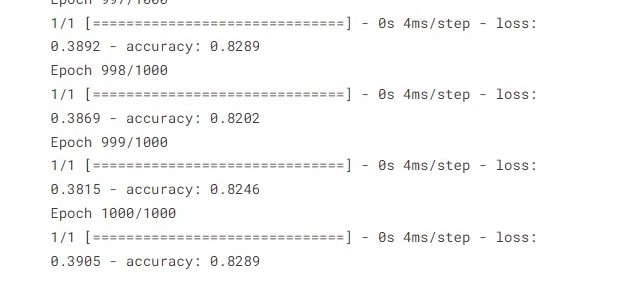`
`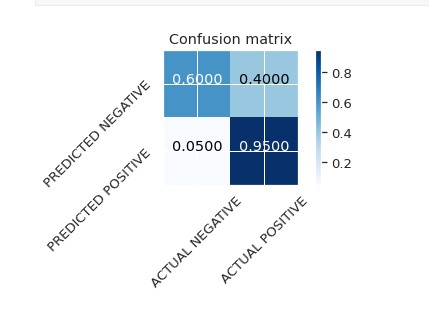`
`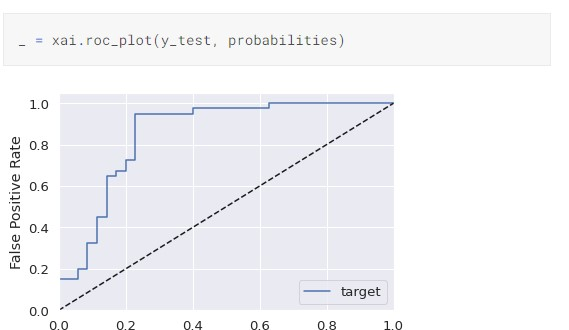`

## Conclusion on Explainable AI

In this article, we figured out a few things related to the topic of XAI, and this tool has recently become of interest to many researchers, data scientists, and analysts. This process is from the beginning and from here comes the benefit of this technology, here in our article we worked on several things, namely the definition of this technology, which as we mentioned is new in this field. We got acquainted with the history of the emergence of this technology and the harm that affected its development, we also defined it and how to use this technology and its advantages and disadvantages, and in the end we applied the code where we fetched the data that was mentioned and worked on it with some tools and then we implemented Xai where it was all mentioned in the above code. In any project, you can see in this attached link and see how this technique works.

I hope you enjoy this article, and then we have several main points, which are that we have clarified the absolute concept behind the technology that we explained in that article, and the second point is understanding the technology and then how it works and what are its different nuclei and what are the features that it consists of and how it serves that technology In the end, we made an implementation of the code that this technology works on.